Question

Use the standard normal table to find the​ z-score that corresponds to the cumulative area 0.0091....

Use the standard normal table to find the​ z-score that corresponds to the cumulative area 0.0091. If the area is not in the​ table, use the entry closest to the area. If the area is halfway between two​ entries, use the​ z-score halfway between the corresponding​ z-scores.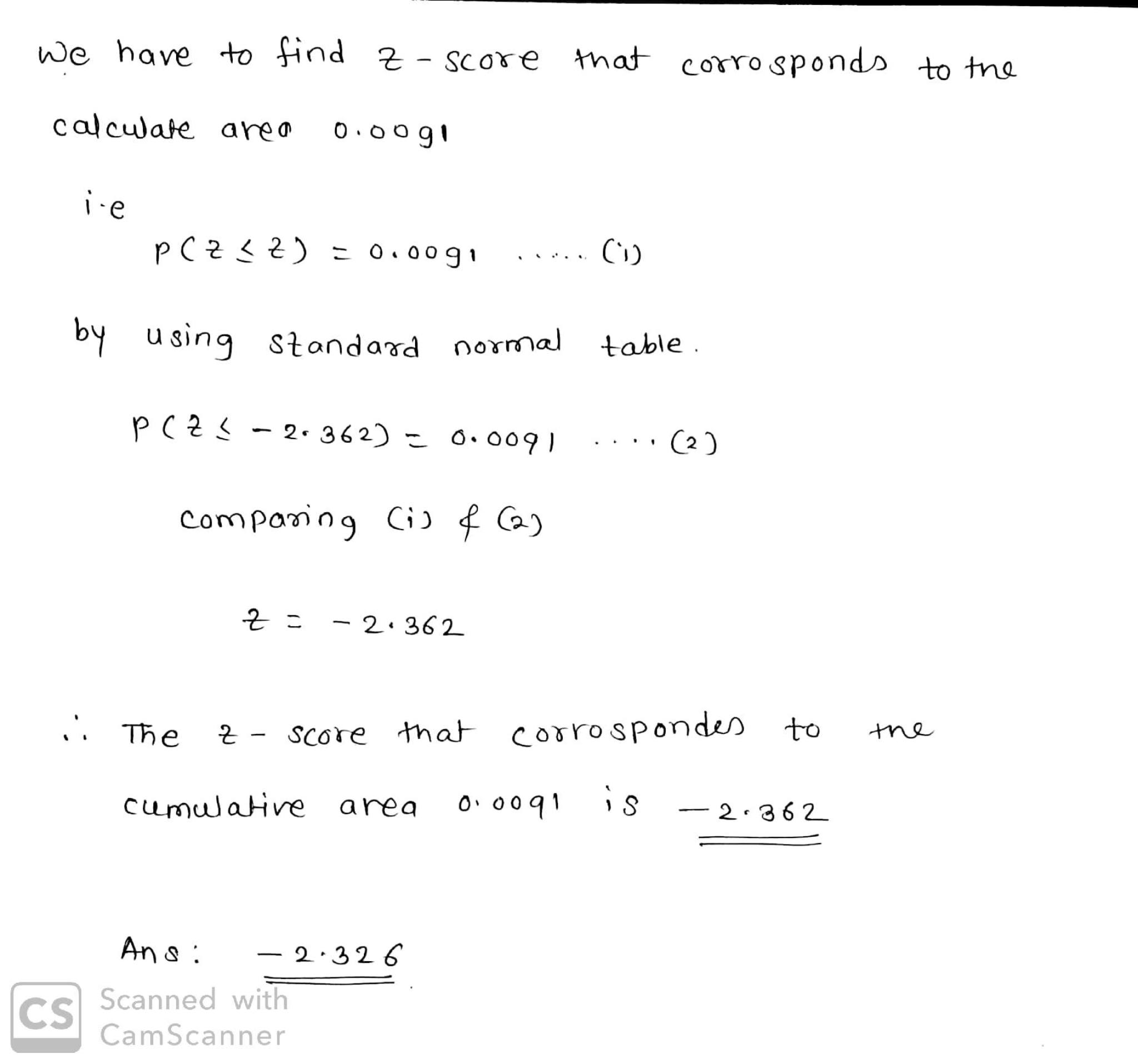Earn Coins

Coins can be redeemed for fabulous gifts.

Similar Homework Help Questions
• Use a table of cumulative areas under the normal curve to find the z-score that corresponds to the given cumulative area

Use a table of cumulative areas under the normal curve to find the z-score that corresponds to the given cumulative area. If the area is not in the table, use the entry closest to the area. If the area is halfway between two entries, use the z-score halfway between the corresponding z-scores. If convenient, use technology to find the z-score.0.049The cumulative area corresponds to the z-score of _______

• Use a table of cumulative areas under the normal curve to find the z-score that corresponds to the given cumulative area

Use a table of cumulative areas under the normal curve to find the z-score that corresponds to the given cumulative area. If the area is not in the table, use the entry closest to the area. If the area is halfway between two entries, use the z-score halfway between the corresponding z-scores. If convenient, use technology to find the z-score.0.053Click to view page 1 of the table. Click to view page 2 of the table.The cumulative area corresponds to the...

• ... Use the standard normal table to find the 2-score that corresponds to the given percentile....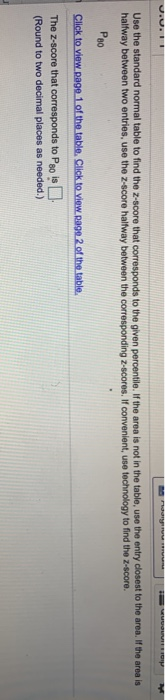... Use the standard normal table to find the 2-score that corresponds to the given percentile. If the area is not in the table, use the entry closest to the area. If the area is halfway between two entries, use the Z-score halfway between the corresponding 2-scores. If convenient, use technology to find the Z-score. Рао Click to view rage 1 of the table. Click to view page 2 of the table. The Z-score that corresponds to Pois (Round to...

• Use the standard normal table to find the Z-score that corresponds to the cumulative area 0.0143....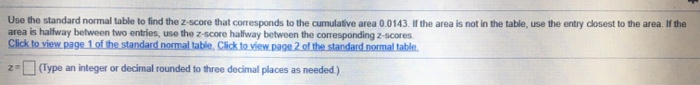Use the standard normal table to find the Z-score that corresponds to the cumulative area 0.0143. If the area is not in the table, use the entry closest to the area. If the area is halfway between two entries, use the z-score halfway between the corresponding 2-scores Click to view page 1 of the standard normal table. Click to view page 2 of the standard normal table. z=(Type an integer or decimal rounded to three decimal places as needed) We...

• Use the Standard Normal Table or technology to find the Z-score that corresponds to the following...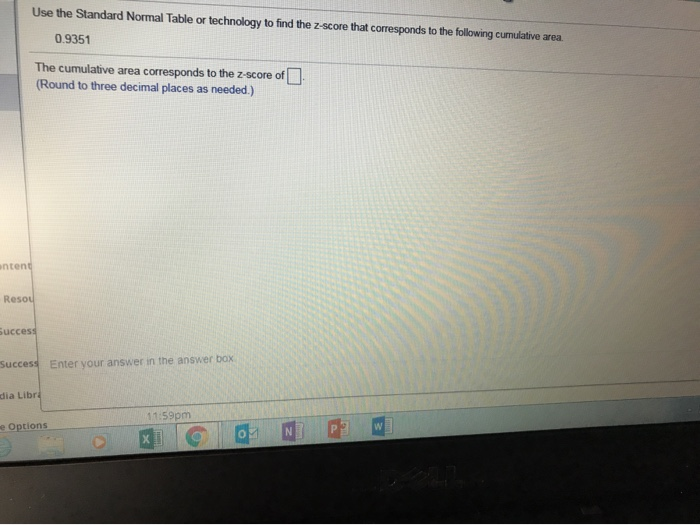Use the Standard Normal Table or technology to find the Z-score that corresponds to the following cumulative area. 0.9351 The cumulative area corresponds to the Z-score of _______

• Use the Standard Normal Table or technology to find the Z-score that corresponds to the following...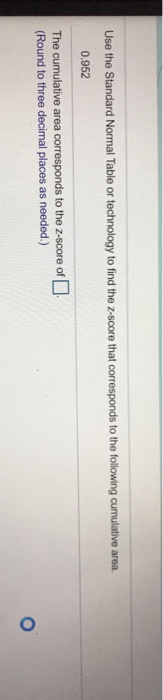Use the Standard Normal Table or technology to find the Z-score that corresponds to the following cumulative area. 0.952 The cumulative area corresponds to the Z-score of (Round to three decimal places as needed.)

• Use the Standard Normal Table or technology to find the Z-score that corresponds to the following...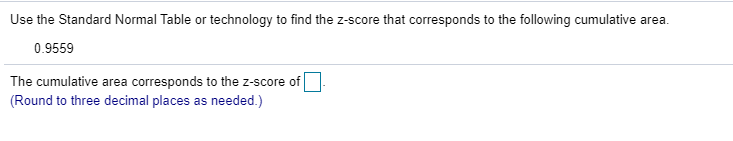Use the Standard Normal Table or technology to find the Z-score that corresponds to the following cumulative area. 0.9559 - The cumulative area corresponds to the Z-score of (Round to three decimal places as needed.)

• Use the Standard Normal Table or technology to find the Z-score that corresponds to the following...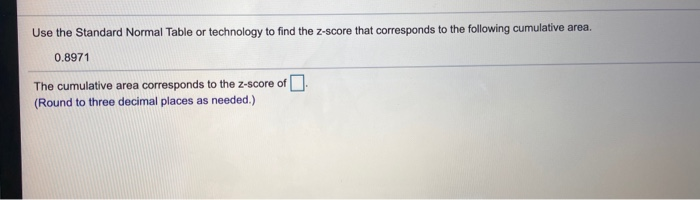Use the Standard Normal Table or technology to find the Z-score that corresponds to the following cumulative area. 0.8971 The cumulative area corresponds to the 2-score of (Round to three decimal places as needed.)

• Use the Cumulative Normal Distribution Table to find the z-score for which the area to its...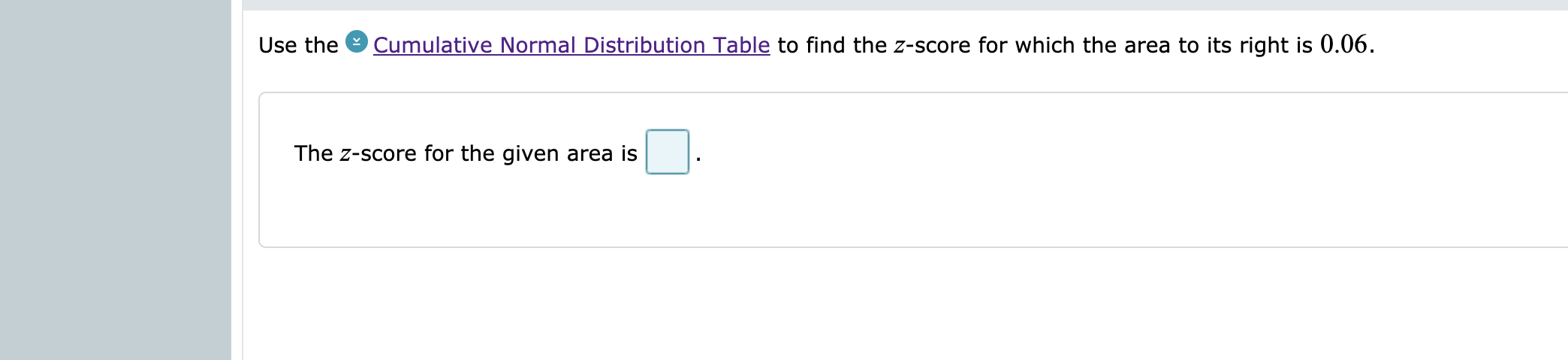Use the Cumulative Normal Distribution Table to find the z-score for which the area to its right is 0.06. The Z-score for the given area is :

• score: of1 p 42 of 8 (0 complete) HW Score: 0%, 0 of 8 pts 5.3.5...score: of1 p 42 of 8 (0 complete) HW Score: 0%, 0 of 8 pts 5.3.5 E Question Help Use a table of cumulative areas under the normal curve to find the z-score that corresponds to the given cumulative area use the entry closest to the area if the area is haltway betvween two use technology to find the z-score If the area is not in the table entries, use the z score haltway between the coresponding z-scores. If convenient...